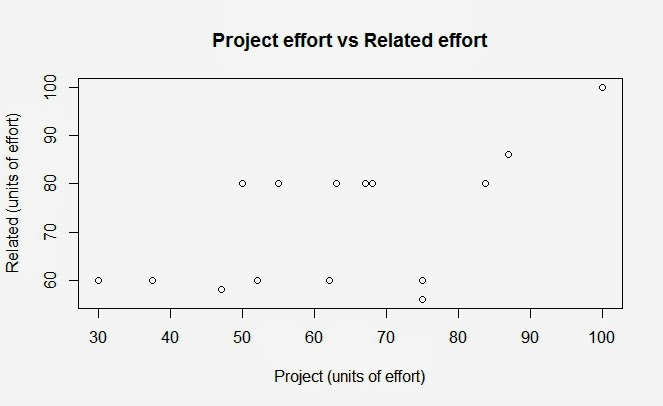## Extreme value detection

There are different interpretations of what constitutes an outlier, one of them is that of extreme values, extreme values are in general localized in the extremes (obviously) and also  some outliers are also located in the extremes , this is not always the case as we can find outliers among clusters of data points, to complicate the scenario even more some outliers can form its own cluster.
I am going to use my log of time (or effort)  used in my own project and related tasks (related only indirectly). I am using two attributes (variables):
1. Effort in project (Effort directly related to the project)
2. Effort in related tasks to the project (Effort related to the project but indirectly).

It is important to note that a score of 100 is almost impossible to achieve as it implies spending more than 18 hours each day working.

I am going to use for this example a common and simple technique for outlier detection or rather extreme value detection.

First, let's see the data (Figure 1),Figure 1. Project and related effort

I think it is not completely clear, but we can see in the upper right corner a  good (maybe not so good) candidate to be considered an outlier (100,100). I will first apply a Z-test ( i know the data doesn't seem normally distributed) to try to identify that outlier, i am using the usual formula Z=|Xi-u|/sd. After that i use other  formula:
f(x1,x2)=|x1-x2|
to test the two dimensions of our data, we can see the result in Figure 2:Fijure 2. Z scores two dimensions

The observation with index 14 it is only aprox. 1.7 deviations above the mean, a common rule is to mark as outliers those scores above 3 deviations. Well then this test only tell us that the observation least inlier (relative to the rest of the points) is the point located in: 100,100. However it is only 1.7 SD above the mean and can ( and maybe should) be considered as normal data.

In future post i will try to apply more advanced outlier detection techniques.